Theory and Modern Applications

# Estimation on Certain Nonlinear Discrete Inequality and Applications to Boundary Value Problem

## Abstract

We investigate certain sum-difference inequalities in two variables which provide explicit bounds on unknown functions. Our result enables us to solve those discrete inequalities considered by Sheng and Li (2008). Furthermore, we apply our result to a boundary value problem of a partial difference equation for estimation.

## 1. Introduction

Various generalizations of the Gronwall inequality [1, 2] are fundamental tools in the study of existence, uniqueness, boundedness, stability, invariant manifolds, and other qualitative properties of solutions of differential equations and integral equation. There are a lot of papers investigating them (such as ). Along with the development of the theory of integral inequalities and the theory of difference equations, more attentions are paid to some discrete versions of Gronwall-Bellman-type inequalities (such as ). Some recent works can be found, for example, in  and some references therein.

We first introduce two lemmas which are useful in our main result.

Lemma 1.1 (the Bernoulli inequality ).

Letand, then.

Lemma 1.2 ().

Assume thatare nonnegative functions andis nonincreasing for all natural numbers, if for all natural numbers,(1.1)

then for all natural numbers,(1.2)

Sheng and Li  considered the inequalities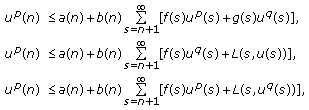(1.3)

wherefor.

In this paper, we investigate certain new nonlinear discrete inequalities in two variables:(1.4)(1.5)(1.6)

wherefor.

Furthermore, we apply our result to a boundary value problem of a partial difference equation for estimation. Our paper gives, in some sense, an extension of a result of .

## 2. Main Result

Throughout this paper, letdenote the set of all real numbers, letbe the given subset of, anddenote the set of nonnegative integers. For functions, their first-order differences are defined by,, and. We use the usual conventions that empty sums and products are taken to be 0 and 1, respectively. In what follows, we assume all functions which appear in the inequalities to be real-value,andare constants, and.

Lemma 2.1.

Assume thatare nonnegative functions defined for, andis nonincreasing in each variable, if(2.1)

then(2.2)

Proof.

Define a functionby(2.3)

The functionis nonincreasing in each variable, so is, we have(2.4)

Using Lemma 1.2, the desired inequality (2.2) is obtained from (2.1), (2.3), and (2.4). This completes the proof of Lemma 2.1.

Theorem 2.2.

Suppose thatandare nonnegative functions defined for,satisfies the inequality (1.4). Then(2.5)

where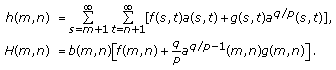(2.6)

Proof.

Define a functionby(2.7)

From (1.4), we have(2.8)

By applying Lemma 1.1, from (2.8), we obtain(2.9)(2.10)

It follows from (2.9) and (2.10) that(2.11)

where we note the definitions ofandin (2.6). From (2.6), we seeis nonnegative and nonincreasing in each variable. By applying Lemma 2.1, the desired inequality (3.3) is obtained from (2.9) and (2.11). This completes the proof of Theorem 2.2.

Theorem 2.3.

Suppose that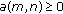andare nonnegative functions defined for,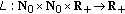satisfies(2.12)

where, andsatisfies the inequality (1.5). Then(2.13)

where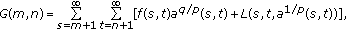(2.14)(2.15)

Proof.

Define a functionby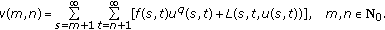(2.16)

Then, as in the proof of Theorem 2.2, we have (2.8), (2.9), and (2.10). By (2.12),(2.17)

It follows from (2.8), (2.9), (2.10), and (2.17) that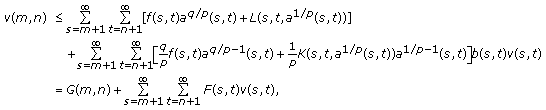(2.18)

where we note the definitions ofand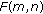in (2.14) and (2.15). From (2.14) we seeis nonnegative and nonincreasing in each variable. By applying Lemma 2.1, the desired inequality (2.19) is obtained from (2.9) and (2.18). This completes the proof of Theorem 2.3.

Theorem 2.4.

Suppose that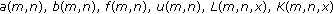are the same as in Theorem 2.3,satisfies the inequality (1.6). Then(2.19)

where(2.20)(2.21)

Proof.

Define a functionby(2.22)

Then, as in the proof of Theorem 2.2, we have (2.8), (2.9), and (2.10). By (2.12),(2.23)

It follows from (2.8), (2.9), (2.10), and (2.23) that(2.24)

whereandare defined by (2.20) and (2.21), respectively. From (2.20), we seeis nonnegative and nonincreasing in each variable. By applying Lemma 2.1, the desired inequality (2.19) is obtained from (2.9) and (2.24). This completes the proof of Theorem 2.4.

## 3. Applications to Boundary Value Problem

In this section, we apply our result to the following boundary value problem (simply called BVP) for the partial difference equation:(3.1)satisfies(3.2)

whereandare constants,, functionsare given, and functionsare nonincreasing. In what follows, we apply our main result to give an estimation of solutions of (3.1).

Corollary 3.1.

All solutionsof BVP (3.1) have the estimate(3.3)

where(3.4)

Proof.

Clearly, the difference equation of BVP (3.1) is equivalent to(3.5)

It follows from (3.2) and (3.5) that(3.6)

Let. Equation (3.6) is of the form (1.4), here. Applying our Theorem 2.2 to inequality (3.6), we obtain the estimate ofas given in Corollary 3.1.

## References

1. Bellman R: The stability of solutions of linear differential equations. Duke Mathematical Journal 1943,10(4):643–647. 10.1215/S0012-7094-43-01059-2

2. Gronwall TH: Note on the derivatives with respect to a parameter of the solutions of a system of differential equations. Annals of Mathematics 1919,20(4):292–296. 10.2307/1967124

3. Agarwal RP, Deng S, Zhang W: Generalization of a retarded Gronwall-like inequality and its applications. Applied Mathematics and Computation 2005,165(3):599–612. 10.1016/j.amc.2004.04.067

4. Lipovan O: Integral inequalities for retarded Volterra equations. Journal of Mathematical Analysis and Applications 2006,322(1):349–358. 10.1016/j.jmaa.2005.08.097

5. Ma Q-H, Yang E-H: On some new nonlinear delay integral inequalities. Journal of Mathematical Analysis and Applications 2000,252(2):864–878. 10.1006/jmaa.2000.7134

6. Pachpatte BG: Inequalities for Differential and Integral Equations, Mathematics in Science and Engineering. Volume 197. Academic Press, San Diego, Calif, USA; 1998:x+611.

7. Wang W-S: A generalized sum-difference inequality and applications to partial difference equations. Advances in Difference Equations 2008, Article ID 695495, 2008:-12.

8. Zhang W, Deng S: Projected Gronwall-Bellman's inequality for integrable functions. Mathematical and Computer Modelling 2001,34(3–4):393–402. 10.1016/S0895-7177(01)00070-X

9. Hull TE, Luxemburg WAJ: Numerical methods and existence theorems for ordinary differential equations. Numerische Mathematik 1960,2(1):30–41. 10.1007/BF01386206

10. Pachpatte BG, Deo SG: Stability of discrete-time systems with retarded argument. Utilitas Mathematica 1973, 4: 15–33.

11. Willett D, Wong JSW: On the discrete analogues of some generalizations of Gronwall's inequality. Monatshefte für Mathematik 1965, 69: 362–367. 10.1007/BF01297622

12. Cheung W-S, Ren J: Discrete non-linear inequalities and applications to boundary value problems. Journal of Mathematical Analysis and Applications 2006,319(2):708–724. 10.1016/j.jmaa.2005.06.064

13. Li WN, Sheng W: Some nonlinear integral inequalities on time scales. Journal of Inequalities and Applications 2007, Article ID 70465, 2007:-15.

14. Pachpatte BG: On some new inequalities related to certain inequalities in the theory of differential equations. Journal of Mathematical Analysis and Applications 1995,189(1):128–144. 10.1006/jmaa.1995.1008

15. Pang PYH, Agarwal RP: On an integral inequality and its discrete analogue. Journal of Mathematical Analysis and Applications 1995,194(2):569–577. 10.1006/jmaa.1995.1318

16. Sheng W, Li WN: Bounds on certain nonlinear discrete inequalities. Journal of Mathematical Inequalities 2008,2(2):279–286.

17. Wang W-S, Shen C-X: On a generalized retarded integral inequality with two variables. Journal of Inequalities and Applications 2008, Article ID 518646, 2008:-9.

18. Mitrinović DS: Analytic Inequalities, Die Grundlehren der Mathematischen Wissenschaften. Volume 16. Springer, New York, NY, USA; 1970:xii+400.

19. Pachpatte BG: On some fundamental finite difference inequalities. Tamkang Journal of Mathematics 2001,32(3):217–223.

## Acknowledgments

This work is supported by Scientific Research Foundation of the Education Department Guangxi Province of China (200707MS112) and by Foundation of Natural Science and Key Discipline of Applied Mathematics of Hechi University of China.

## Author information

Authors

### Corresponding author

Correspondence to Wu-Sheng Wang.

## Rights and permissions

Reprints and Permissions

Wang, WS. Estimation on Certain Nonlinear Discrete Inequality and Applications to Boundary Value Problem. Adv Differ Equ 2009, 708587 (2009). https://doi.org/10.1155/2009/708587